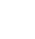# Excel Multiple Child SKUs

Our clients product list was provided to them in Excel format but each product had a Child SKU labelled across the row, sometimes up to 200 individual SKU codes.This data needed to be structured differently in order to be updated to the clients website correctly and to do this job manually would have taken in excess of 50 person-hours cutting and pasting. It was an extremely laborious task.

Our brief was to create a code that could achieve this desired output in a very quick time. The following code was applied to the workbook and the desired output was obtained in under 10 seconds.

The code can be adapted however you see fit, and we would recommend using the lastrow and lastcol VBA that are shown in this datanology blog.

``` Sub Button1_Click()```

``` Application.ScreenUpdating = False 'Set Output row to as first to populate OTP = 2 'traverse all the data range and if not blank take it over as new child SKU For r = 2 To 1053 Application.StatusBar = r For c = 5 To 255 If ActiveSheet.Cells(r, c) <> "" Then Worksheets("Output").Range("A" & OTP) = ActiveSheet.Range("A" & r) Worksheets("Output").Range("B" & OTP) = ActiveSheet.Range("B" & r) Worksheets("Output").Range("C" & OTP) = ActiveSheet.Range("C" & r) Worksheets("Output").Range("D" & OTP) = ActiveSheet.Range("D" & r) Worksheets("Output").Range("E" & OTP) = ActiveSheet.Cells(r, c) 'Increment output row by 1 OTP = OTP + 1 End If Next c Next r ```